Anzeige

# 09 UNIT-9(Electronics and down of Modern Physics) (1).pptx

23. Mar 2023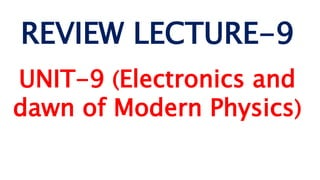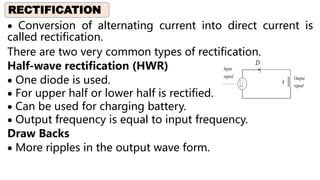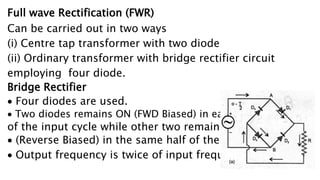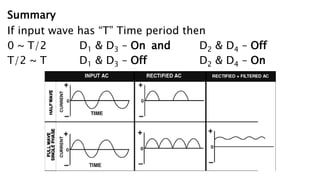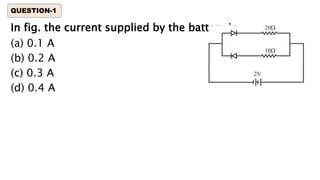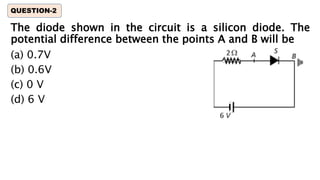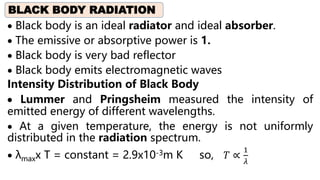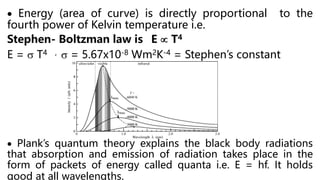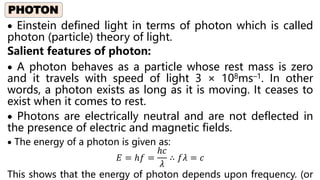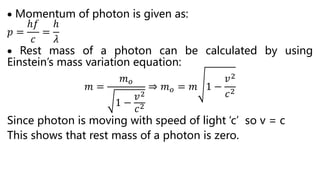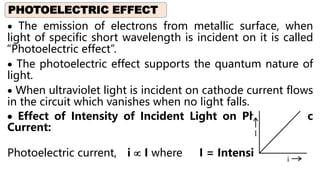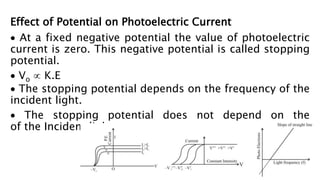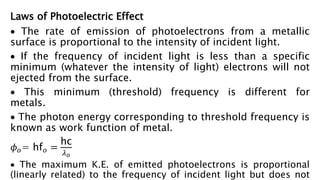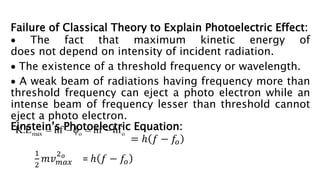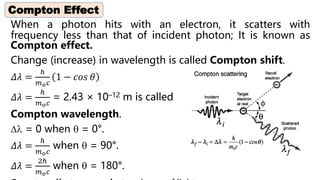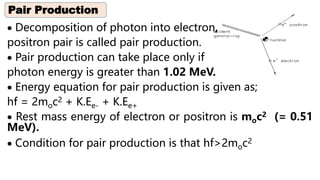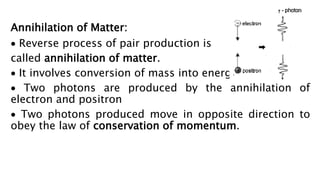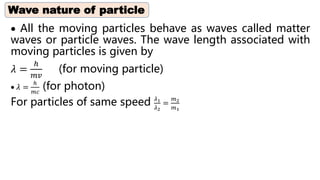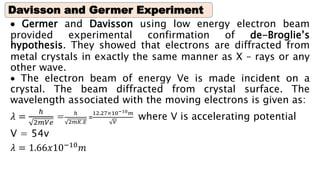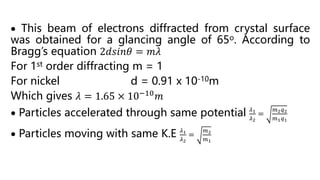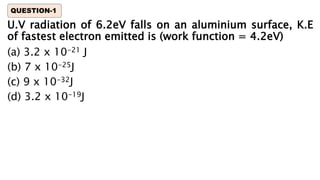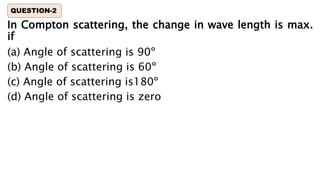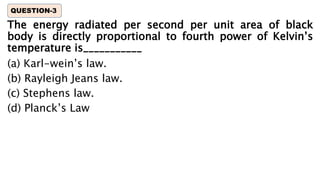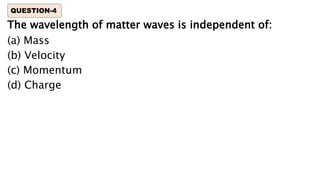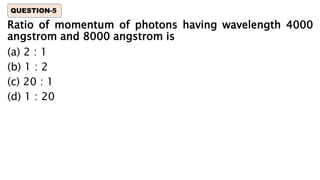1 von 25
Anzeige

### 09 UNIT-9(Electronics and down of Modern Physics) (1).pptx

1. REVIEW LECTURE-9 UNIT-9 (Electronics and dawn of Modern Physics)
2.  Conversion of alternating current into direct current is called rectification. There are two very common types of rectification. Half-wave rectification (HWR)  One diode is used.  For upper half or lower half is rectified.  Can be used for charging battery.  Output frequency is equal to input frequency. Draw Backs  More ripples in the output wave form. RECTIFICATION D Output signal Input signal R
3. Full wave Rectification (FWR) Can be carried out in two ways (i) Centre tap transformer with two diode (ii) Ordinary transformer with bridge rectifier circuit employing four diode. Bridge Rectifier  Four diodes are used.  Two diodes remains ON (FWD Biased) in each half of the input cycle while other two remains off  (Reverse Biased) in the same half of the cycle.  Output frequency is twice of input frequency.
4. Summary If input wave has “T” Time period then 0 ~ T/2 D1 & D3 – On and D2 & D4 – Off T/2 ~ T D1 & D3 – Off D2 & D4 – On
5. In fig. the current supplied by the battery is (a) 0.1 A (b) 0.2 A (c) 0.3 A (d) 0.4 A QUESTION-1
6. The diode shown in the circuit is a silicon diode. The potential difference between the points A and B will be (a) 0.7V (b) 0.6V (c) 0 V (d) 6 V QUESTION-2
7.  Black body is an ideal radiator and ideal absorber.  The emissive or absorptive power is 1.  Black body is very bad reflector  Black body emits electromagnetic waves Intensity Distribution of Black Body  Lummer and Pringsheim measured the intensity of emitted energy of different wavelengths.  At a given temperature, the energy is not uniformly distributed in the radiation spectrum.  λmaxx T = constant = 2.9x10-3m K so, 𝑇 ∝ 1 𝜆 BLACK BODY RADIATION
8.  Energy (area of curve) is directly proportional to the fourth power of Kelvin temperature i.e. Stephen- Boltzman law is E  T4 E =  T4 ,  = 5.67x10-8 Wm2K-4 = Stephen’s constant  Plank’s quantum theory explains the black body radiations that absorption and emission of radiation takes place in the form of packets of energy called quanta i.e. E = hf. It holds good at all wavelengths.
9.  Einstein defined light in terms of photon which is called photon (particle) theory of light. Salient features of photon:  A photon behaves as a particle whose rest mass is zero and it travels with speed of light 3 × 108ms–1. In other words, a photon exists as long as it is moving. It ceases to exist when it comes to rest.  Photons are electrically neutral and are not deflected in the presence of electric and magnetic fields.  The energy of a photon is given as: 𝐸 = ℎ𝑓 = ℎ𝑐 𝜆 ∴ 𝑓𝜆 = 𝑐 This shows that the energy of photon depends upon frequency. (or PHOTON
10.  Momentum of photon is given as: 𝑝 = ℎ𝑓 𝑐 = ℎ 𝜆  Rest mass of a photon can be calculated by using Einstein’s mass variation equation: 𝑚 = 𝑚𝑜 1 − 𝑣2 𝑐2 ⇒ 𝑚𝑜 = 𝑚 1 − 𝑣2 𝑐2 Since photon is moving with speed of light ‘c’ so v = c This shows that rest mass of a photon is zero.
11.  The emission of electrons from metallic surface, when light of specific short wavelength is incident on it is called “Photoelectric effect”.  The photoelectric effect supports the quantum nature of light.  When ultraviolet light is incident on cathode current flows in the circuit which vanishes when no light falls.  Effect of Intensity of Incident Light on Photoelectric Current: Photoelectric current, i  I where I = Intensity of light PHOTOELECTRIC EFFECT
12. Effect of Potential on Photoelectric Current  At a fixed negative potential the value of photoelectric current is zero. This negative potential is called stopping potential.  Vo  K.E  The stopping potential depends on the frequency of the incident light.  The stopping potential does not depend on the of the Incident light.
13. Laws of Photoelectric Effect  The rate of emission of photoelectrons from a metallic surface is proportional to the intensity of incident light.  If the frequency of incident light is less than a specific minimum (whatever the intensity of light) electrons will not ejected from the surface.  This minimum (threshold) frequency is different for metals.  The photon energy corresponding to threshold frequency is known as work function of metal. 𝜙𝑜= hf𝑜 = hc 𝜆𝑜  The maximum K.E. of emitted photoelectrons is proportional (linearly related) to the frequency of incident light but does not
14. Failure of Classical Theory to Explain Photoelectric Effect:  The fact that maximum kinetic energy of does not depend on intensity of incident radiation.  The existence of a threshold frequency or wavelength.  A weak beam of radiations having frequency more than threshold frequency can eject a photo electron while an intense beam of frequency lesser than threshold cannot eject a photo electron. Einstein’s Photoelectric Equation: = ℎ 𝑓 − 𝑓𝑜 1 2 𝑚𝑣𝑚𝑎𝑥 2𝑜 = ℎ 𝑓 − 𝑓𝑜 max o o K.E hf hf hf    
15. When a photon hits with an electron, it scatters with frequency less than that of incident photon; It is known as Compton effect. Change (increase) in wavelength is called Compton shift. 𝛥𝜆 = ℎ 𝑚𝑜𝑐 1 − 𝑐𝑜𝑠 𝜃 𝛥𝜆 = ℎ 𝑚𝑜𝑐 = 2.43 × 10–12 m is called Compton wavelength.  = 0 when  = 0°. 𝛥𝜆 = ℎ 𝑚𝑜𝑐 when  = 90°. 𝛥𝜆 = 2ℎ 𝑚𝑜𝑐 when  = 180°. Compton Effect
16.  Decomposition of photon into electron, positron pair is called pair production.  Pair production can take place only if photon energy is greater than 1.02 MeV.  Energy equation for pair production is given as; hf = 2moc2 + K.Ee- + K.Ee+  Rest mass energy of electron or positron is moc2 (= 0.51 MeV).  Condition for pair production is that hf>2moc2 Pair Production
17. Annihilation of Matter:  Reverse process of pair production is called annihilation of matter.  It involves conversion of mass into energy.  Two photons are produced by the annihilation of electron and positron  Two photons produced move in opposite direction to obey the law of conservation of momentum.
18.  All the moving particles behave as waves called matter waves or particle waves. The wave length associated with moving particles is given by 𝜆 = ℎ 𝑚𝑣 (for moving particle)  𝜆 = ℎ 𝑚𝑐 (for photon) For particles of same speed 𝜆1 𝜆2 = 𝑚2 𝑚1 Wave nature of particle
19.  Germer and Davisson using low energy electron beam provided experimental confirmation of de-Broglie’s hypothesis. They showed that electrons are diffracted from metal crystals in exactly the same manner as X – rays or any other wave.  The electron beam of energy Ve is made incident on a crystal. The beam diffracted from crystal surface. The wavelength associated with the moving electrons is given as: 𝜆 = ℎ 2𝑚𝑉𝑒 = ℎ 2𝑚𝐾.𝐸 = 12.27×10−10𝑚 𝑉 where V is accelerating potential V = 54v 𝜆 = 1.66𝑥10−10 𝑚 Davisson and Germer Experiment
20.  This beam of electrons diffracted from crystal surface was obtained for a glancing angle of 65o. According to Bragg’s equation 2𝑑𝑠𝑖𝑛𝜃 = 𝑚𝜆 For 1st order diffracting m = 1 For nickel d = 0.91 x 10-10m Which gives 𝜆 = 1.65 × 10−10 𝑚  Particles accelerated through same potential 𝜆1 𝜆2 = 𝑚2𝑞2 𝑚1𝑞1  Particles moving with same K.E 𝜆1 𝜆2 = 𝑚2 𝑚1
21. U.V radiation of 6.2eV falls on an aluminium surface, K.E of fastest electron emitted is (work function = 4.2eV) (a) 3.2 x 10-21 J (b) 7 x 10-25J (c) 9 x 10-32J (d) 3.2 x 10-19J QUESTION-1
22. In Compton scattering, the change in wave length is max. if (a) Angle of scattering is 90º (b) Angle of scattering is 60º (c) Angle of scattering is180º (d) Angle of scattering is zero QUESTION-2
23. The energy radiated per second per unit area of black body is directly proportional to fourth power of Kelvin’s temperature is___________ (a) Karl-wein’s law. (b) Rayleigh Jeans law. (c) Stephens law. (d) Planck’s Law QUESTION-3
24. The wavelength of matter waves is independent of: (a) Mass (b) Velocity (c) Momentum (d) Charge QUESTION-4
25. Ratio of momentum of photons having wavelength 4000 angstrom and 8000 angstrom is (a) 2 : 1 (b) 1 : 2 (c) 20 : 1 (d) 1 : 20 QUESTION-5
Anzeige